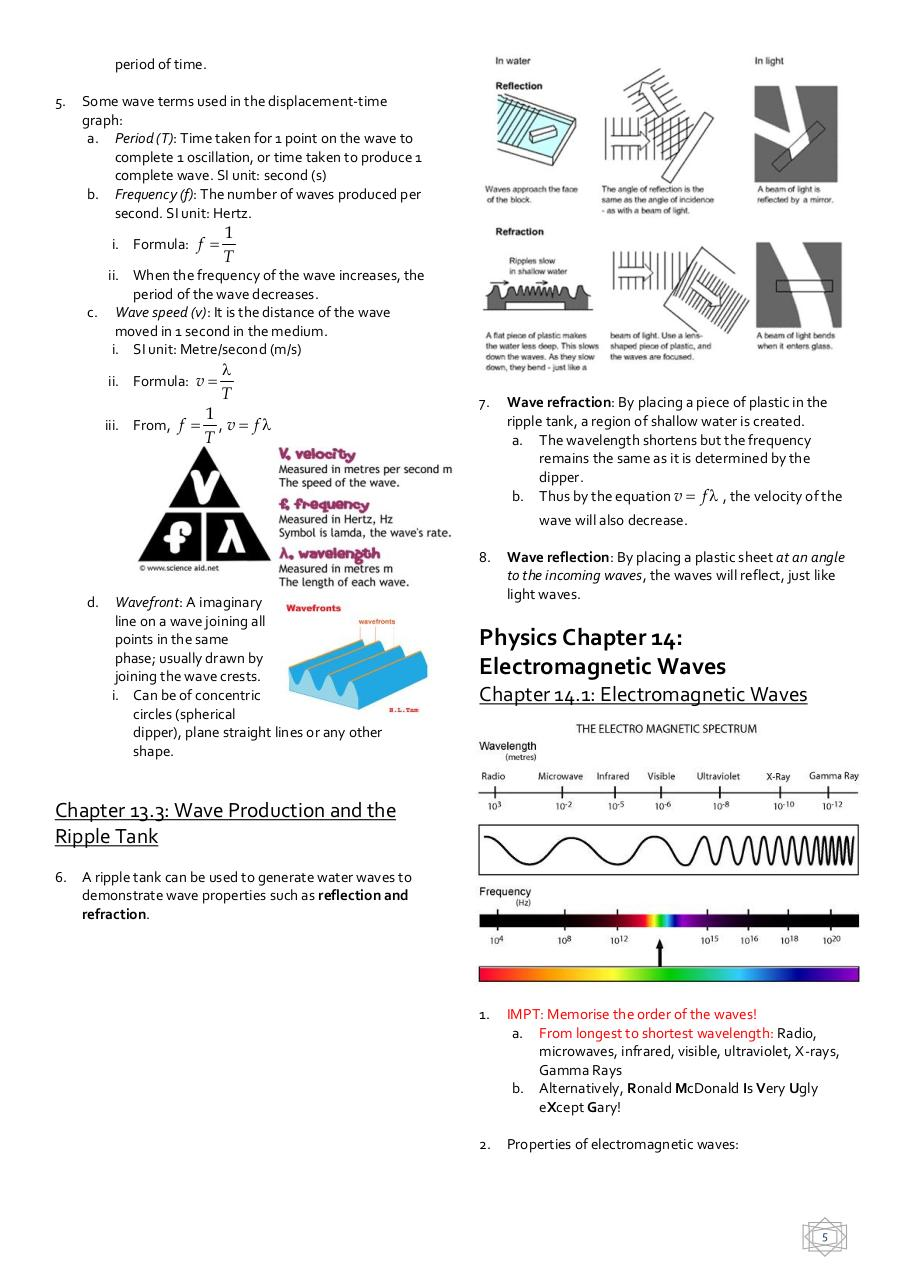# Waves.pdfPage 1 2 3 4 5 6 7

#### Text preview

period of time.
5.

Some wave terms used in the displacement-time
graph:
a. Period (T): Time taken for 1 point on the wave to
complete 1 oscillation, or time taken to produce 1
complete wave. SI unit: second (s)
b. Frequency (f): The number of waves produced per
second. SI unit: Hertz.
i. Formula: f 

1
T

ii. When the frequency of the wave increases, the
period of the wave decreases.
c. Wave speed (v): It is the distance of the wave
moved in 1 second in the medium.
i. SI unit: Metre/second (m/s)
ii. Formula: v 

T

1
iii. From, f  , v  f 
T

7.

Wave refraction: By placing a piece of plastic in the
ripple tank, a region of shallow water is created.
a. The wavelength shortens but the frequency
remains the same as it is determined by the
dipper.
b. Thus by the equation v  f  , the velocity of the
wave will also decrease.

8.
d.

Wavefront: A imaginary
line on a wave joining all
points in the same
phase; usually drawn by
joining the wave crests.
i. Can be of concentric
circles (spherical
dipper), plane straight lines or any other
shape.

Wave reflection: By placing a plastic sheet at an angle
to the incoming waves, the waves will reflect, just like
light waves.

Physics Chapter 14:
Electromagnetic Waves
Chapter 14.1: Electromagnetic Waves

Chapter 13.3: Wave Production and the
Ripple Tank
6.

A ripple tank can be used to generate water waves to
demonstrate wave properties such as reflection and
refraction.

1.

IMPT: Memorise the order of the waves!
a. From longest to shortest wavelength: Radio,
microwaves, infrared, visible, ultraviolet, X-rays,
Gamma Rays
b. Alternatively, Ronald McDonald Is Very Ugly
eXcept Gary!

2.

Properties of electromagnetic waves:

5